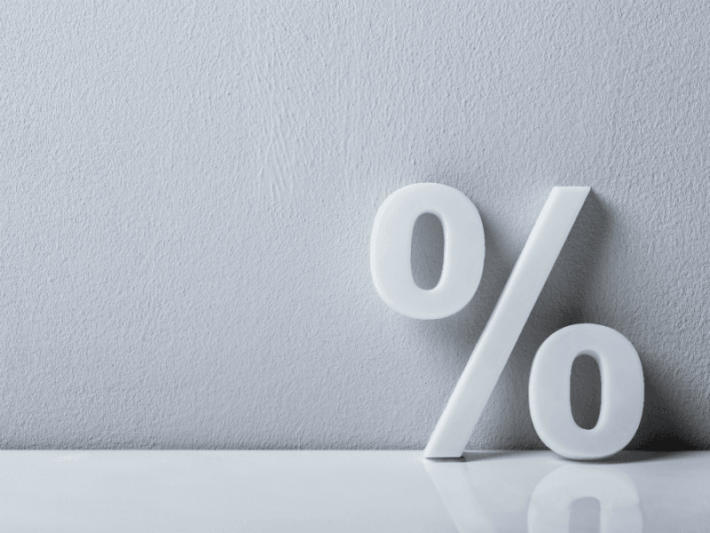ôô

# How to Quickly Calculate PercentagesHow to calculate percentages is easier than you think. Quick, what’s 36% of 25? Or how about 250% of 20? Learn a quick and dirty tip to help you calculate all of those pesky percentages in your head.

By
Jason Marshall, PhD
Episode #127How to calculate percentages can be easier than you may realize. Keep reading for some simple tricks.

Long time math fans may remember our first foray into the world of percentages way back in the 12th and 13th episodes of the podcast. In those shows we learned what percentages are, how they’re related to fractions, how to use percentages to easily calculate tips at restaurants, and how to use percentages to easily calculate sales prices when shopping.

If you’re not sure how to perform any of those handy calculations, or if you’re just in need of a general percentage refresher, I highly recommend taking a look at those earlier shows and getting yourself up to speed. Why? Because once you’re caught up, you’ll be ready to step up and learn how to become a true percentage-calculating machine. Which is exactly what we’re going to turn you into today.

As an Amazon Associate and a Bookshop.org Affiliate, QDT earns from qualifying purchases.

## Recap: What Are Percentages?

To make sure we’re all on the same page, let’s kick things off by taking a minute to recap a few key facts about percentages. Let’s start with the most important question: What are percentages? Perhaps the most illuminating thing to know is that the word “percent” is really just the phrase “per cent” squashed together. And since “cent” here means 100 (as in “century”), we see that the word “percent” just means “per 100.” In other words, 10% means “10 per 100,” which is the same as the fraction 10/100 or 1/10.

This turns out to be great news since it makes lots of percentages easy to calculate. In particular, it’s easy to calculate 10% of any number since that’s just 1/10 of the number. Why is that so helpful? Because it means that you can quickly calculate 10% of a number simply by moving its decimal point 1 position to the left.

But what about calculating something like 36% of 25? Or maybe 250% of 20? In these cases, our trick of using the power of 10% doesn’t help—so what can we do?

## How to Quickly Calculate Percentages

One trick that will often help you quickly calculate these types of percentages is to use the fact that x percent of y is the same as y percent of x. Huh? I know that might sound kind of confusing, but it’s actually pretty simple. Taking our example from before, this rule says that 36% of 25 is the same as 25% of 36. How does that help us? Well, since 25% is the same as the fraction 1/4, we see that 25% of 36 must be 36/4 or 9. So 25% of 36 is equal to 9, and 36% of 25 must also be 9.

The beauty of this trick is that every time you’ve solved one problem, you’ve actually solved two! And that’s especially useful when one of the problems is much easier to solve than the other—as was the case here.

It’s helpful to take a minute to see why this seemingly magical trick works. As I mentioned before, 36% is equivalent to the fraction 36/100. Since we can write the fraction 1/100 as the decimal number 0.01, we see that the fraction 36/100 can also be written 0.01 x 36. That means that 36% of 25 must be equal to (0.01 x 36) x 25. Now here comes the cool part: The associative property of multiplication tells us that we can multiply several numbers in any order we’d like. Which means that (0.01 x 36) x 25 = (0.01 x 25) x 36. But 0.01 x 25 is the same thing as 25%, which means that this is equal to 25% of 36. So 36% of 25 is equal to 25% of 36. It ain’t magic, it’s math! Pretty cool, right?

## Pages